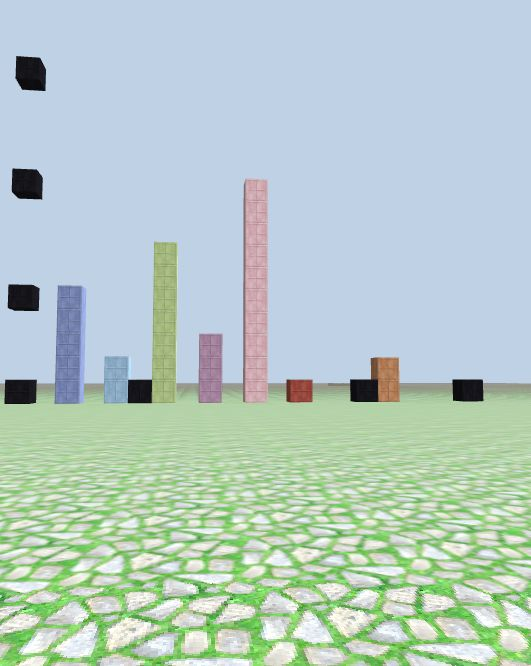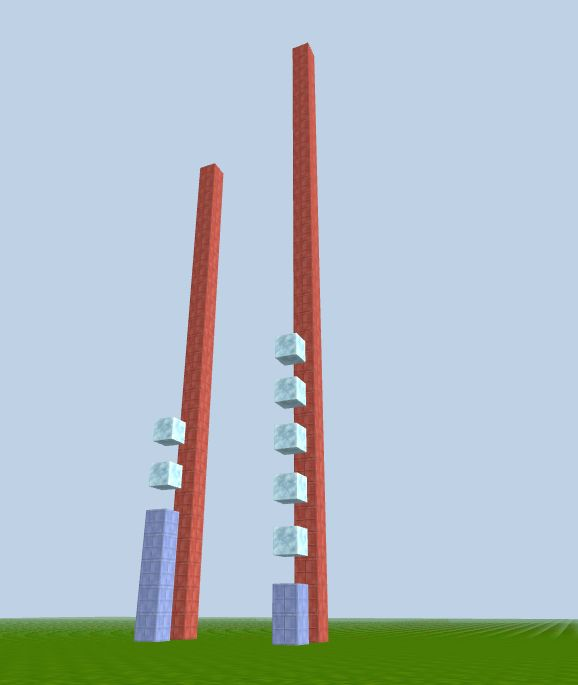# Basic Math Operations

##### JavaScript arithmetic operators

We will take a brief look at some basic math operators and their usage:

Operator Description
`+` Addition
`-` Subtraction
`*` Multiplication
`/` Division
`**` Exponentiation (power)
`%` modulo - returns the remainder of the division
`++` Increment (add 1)
`--` Decrement (subtract 1)

A typical arithmetic operation operates on numbers (literals or variables):

``````// numbers can be literals:
let x = 100 + 50;

// or variables:
let y = x + 10;

// or expressions:
let z = (100 + 50) * x;
``````
##### Math functions

JavaScript has a built-in object `Math` that contains many mathematical constants and functions. For example:

`Math.abs(x)` returns the absolute (positive) value of `x`. `Math.floor(x)` returns the largest integer less than or equal to `x`.

##### Examples
``````console.log(7.0 / 2);                // 3.5
console.log(3 * 3.75 / 1.5);         // 7.5
console.log((3 + 5) * 2 * 3);        // 48
console.log((3 + 4) * (5**2 - 5));   // 140
console.log(13 % 3);                 // 1
console.log(3**2);                   // 9

let k = 3;
let h = 10;
k++;              // increment operator ++
h--;              // decrement operator --
console.log(k);           // 4
console.log(h);           // 9
``````

### Learn with CodeCraft visual

``````// basic math operations
let t = 5;
let s = 2;

// columns use the math results as the heights:
column_m(2, -20, t, 2)
column_m(4, -20, s, 7)

column_m(6, -20, t + s, 8)
column_m(8, -20, t - s, 12)
column_m(10, -20, t * s, 11)
``````
##### modulo

`%` returns the remainder of the division, red block at at `x = 5%2 = 1, y=3, z=-20`

``````// red column shows the modulo result 5%2=1
column_m(12, -20, t % s, 13)      // red
``````
##### Division, Math.floor()

`t/s` might return a floating point result, we can use a Math object method, `Math.floor()`

`Math.floor()` returns the whole number part:

``````// orange, Math.floor(t/s)
column_m(16, -20, Math.floor(t/s), 10)      // orange, 2
``````##### power

The blue columns in the next picture represent the base number, and the diamond blocks above the column represent the power it is raised to. The red column behind the column indicates the result of the power calculation.

``````// 5**2
column_m(2, -20, t, 2);     // base number t, blue

for (let j = 0; j < s; j++) {
block_m(2, (t+2+j*2), -20, 19);    // diamond blocks, the power s

column_m(2, -22, t**s, 13);   // 5**2, the red column has height 5**2=25

// try 2**5
column_m(8, -20, s, 2);    // base number s= 2, blue

for (let j = 0; j < t; j++) {
block_m(8,(2*j+s+2), -20, 19);     // diamonds, power t

column_m(8, -22, s**t, 13)   // 2**5 the red column has height 2**5=32
``````

left: 5**2; right: 2**5##### Math.abs()

`Math.abs(x)` returns the absolute (positive) value of `x`:

``````let x1 = -2, x2 = -4, x3 = -5, x4 = -1;

// blue blocks at x1 ... x4, negative locations
block_m(x1, 2, -20, 45);
block_m(x2, 3, -20, 45);
block_m(x3, 4, -20, 45);
block_m(x4, 6, -20, 45);

// red blocks at positive side abs(x1)...abs(x4)
block_m(Math.abs(x1), 2, -20, 56);
block_m(Math.abs(x2), 3, -20, 56);
block_m(Math.abs(x3), 4, -20, 56);
block_m(Math.abs(x4), 6, -20, 56);
``````

Red blocks at the positive side mirror the blue blue blocks at the negative side### Compound assignment operators

When writing programs we often need to change the value of an existing variable. For example, in a game the `score` variable is updated whenever the player hits the target:

``````let score = 0;

// ... game logic here

score = score + 20;
``````

This expression is used very frequently, JavaScript (and many other programming languages) has a shorthand for it:

``````score += 20;
``````

Similarly you can use `-=`, `*=` and `/=`.

##### Increment and decrement operators

With compound operator we can increase a variable by 1 using `x += 1`. But there is even a shorter expression to do the same:

``````x++;
``````

Similarly `x--` is the same as `x -= 1`. These operators are often used in loops to keep a count on how many more iterations to run.